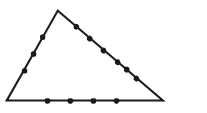# If the sides AB, BC and CA

Question:

If the sides $A B, B C$ and $C A$ of a triangle $A B C$ have 3,5 and 6 interior points respectively, then the total number of triangles that can be constructed using these points as vertices, is equal to :

1. 364

2. 240

3. 333

4. 360

Correct Option: , 3

Solution:Total Number of triangles formed

$={ }^{14} \mathrm{C}_{3}-{ }^{3} \mathrm{C}_{3}-{ }^{5} \mathrm{C}_{3}-{ }^{6} \mathrm{C}_{3}$

$=333$